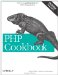# Recipe 2.6. Generating Biased Random Numbers

#### 2.6.1. Problem

You want to generate random numbers, but you want these numbers to be somewhat biased, so that numbers in certain ranges appear more frequently than others. For example, you want to spread out a series of banner ad impressions in proportion to the number of impressions remaining for each ad campaign.

#### 2.6.2. Solution

Use the pc_rand_weighted( ) function shown in Example 2-2.

##### pc_rand_weighted( )

 ` \$weight) { \$total += \$weight; \$distribution[\$number] = \$total; } \$rand = mt_rand(0, \$total - 1); foreach (\$distribution as \$number => \$weights) { if (\$rand < \$weights) { return \$number; } } } ?>`

#### 2.6.3. Discussion

Imagine if instead of an array in which the values are the number of remaining impressions, you have an array of ads in which each ad occurs exactly as many times as its remaining number of impressions. You can simply pick an unweighted random place within the array, and that'd be the ad that shows.

This technique can consume a lot of memory if you have millions of impressions remaining. Instead, you can calculate how large that array would be (by totaling the remaining impressions), pick a random number within the size of the make-believe array, and then go through the array figuring out which ad corresponds to the number you picked. For instance:

`\$ads = array('ford' => 12234, // advertiser, remaining impressions              'att'  => 33424,              'ibm'  => 16823); \$ad = pc_rand_weighted(\$ads);`

Recipe 2.5 for how to generate random numbers within a range .PHP Cookbook: Solutions and Examples for PHP Programmers
ISBN: 0596101015
EAN: 2147483647
Year: 2006
Pages: 445

Similar book on Amazon